Chapter 5. Stoichiometry and the Mole

# Mole-Mass and Mass-Mass Calculations

Learning Objectives

1. From a given number of moles of a substance, calculate the mass of another substance involved using the balanced chemical equation.
2. From a given mass of a substance, calculate the moles of another substance involved using the balanced chemical equation.
3. From a given mass of a substance, calculate the mass of another substance involved using the balanced chemical equation.

Mole-mole calculations are not the only type of calculations that can be performed using balanced chemical equations. Recall that the molar mass can be determined from a chemical formula and used as a conversion factor. We can add that conversion factor as another step in a calculation to make a , where we start with a given number of moles of a substance and calculate the mass of another substance involved in the chemical equation, or vice versa.

For example, suppose we have the balanced chemical equation:

2Al + 3Cl2 → 2AlCl3

Suppose we know we have 123.2 g of Cl2. How can we determine how many moles of AlCl3 we will get when the reaction is complete? First and foremost, chemical equations are not balanced in terms of grams; they are balanced in terms of moles. So to use the balanced chemical equation to relate an amount of Cl2 to an amount of AlCl3, we need to convert the given amount of Cl2 into moles. We know how to do this by simply using the molar mass of Cl2 as a conversion factor. The molar mass of Cl2 (which we get from the atomic mass of Cl from the periodic table) is 70.90 g/mol. We must invert this fraction so that the units cancel properly: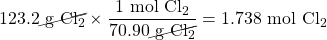Now that we have the quantity in moles, we can use the balanced chemical equation to construct a conversion factor that relates the number of moles of Cl2 to the number of moles of AlCl3. The numbers in the conversion factor come from the coefficients in the balanced chemical equation: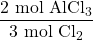Using this conversion factor with the molar quantity we calculated above, we get: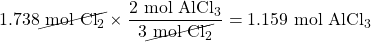So, we will get 1.159 mol of AlCl3 if we react 123.2 g of Cl2.

In this last example, we did the calculation in two steps. However, it is mathematically equivalent to perform the two calculations sequentially on one line: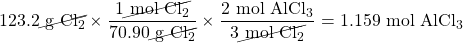The units still cancel appropriately, and we get the same numerical answer in the end. Sometimes the answer may be slightly different from doing it one step at a time because of rounding of the intermediate answers, but the final answers should be effectively the same.

Example 5.8

# Problem

How many moles of HCl will be produced when 249 g of AlCl3 are reacted according to this chemical equation?

2AlCl3 + 3H2O(ℓ) → Al2O3 + 6HCl(g)

## Solution

We will do this in two steps: convert the mass of AlCl3 to moles and then use the balanced chemical equation to find the number of moles of HCl formed. The molar mass of AlCl3 is 133.33 g/mol, which we have to invert to get the appropriate conversion factor: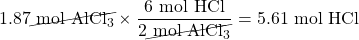Now we can use this quantity to determine the number of moles of HCl that will form. From the balanced chemical equation, we construct a conversion factor between the number of moles of AlCl3 and the number of moles of HCl: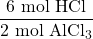Applying this conversion factor to the quantity of AlCl3, we get:Alternatively, we could have done this in one line: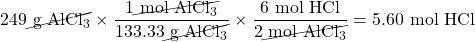The last digit in our final answer is slightly different because of rounding differences, but the answer is essentially the same.

# Test Yourself

How many moles of Al2O3 will be produced when 23.9 g of H2O are reacted according to this chemical equation?

2AlCl3 + 3H2O(ℓ) → Al2O3 + 6HCl(g)

0.442 mol

A variation of the mole-mass calculation is to start with an amount in moles and then determine an amount of another substance in grams. The steps are the same but are performed in reverse order.

Example 5.9

# Problem

How many grams of NH3 will be produced when 33.9 mol of H2 are reacted according to this chemical equation?

N2(g) + 3H2(g) → 2NH3(g)

## Solution

The conversions are the same, but they are applied in a different order. Start by using the balanced chemical equation to convert to moles of another substance and then use its molar mass to determine the mass of the final substance. In two steps, we have: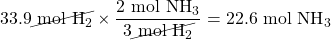Now, using the molar mass of NH3, which is 17.03 g/mol, we get: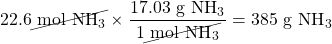# Test Yourself

How many grams of N2 are needed to produce 2.17 mol of NH3 when reacted according to this chemical equation?

N2(g) + 3H2(g) → 2NH3(g)

30.4 g (Note: here we go from a product to a reactant, showing that mole-mass problems can begin and end with any substance in the chemical equation.)

It should be a trivial task now to extend the calculations to , in which we start with a mass of some substance and end with the mass of another substance in the chemical reaction. For this type of calculation, the molar masses of two different substances must be used—be sure to keep track of which is which. Again, however, it is important to emphasize that before the balanced chemical reaction is used, the mass quantity must first be converted to moles. Then the coefficients of the balanced chemical reaction can be used to convert to moles of another substance, which can then be converted to a mass.

For example, let us determine the number of grams of SO3 that can be produced by the reaction of 45.3 g of SO2 and O2:

2SO2(g) + O2(g) → 2SO3(g)

First, we convert the given amount, 45.3 g of SO2, to moles of SO2 using its molar mass (64.06 g/mol):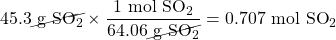Second, we use the balanced chemical reaction to convert from moles of SO2 to moles of SO3: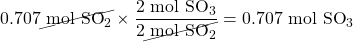Finally, we use the molar mass of SO3 (80.06 g/mol) to convert to the mass of SO3: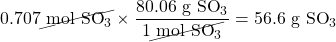We can also perform all three steps sequentially, writing them on one line as: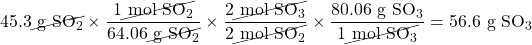We get the same answer. Note how the initial and all the intermediate units cancel, leaving grams of SO3, which is what we are looking for, as our final answer.

Example 5.10

# Problem

What mass of Mg will be produced when 86.4 g of K are reacted?

MgCl2(s) + 2K(s) → Mg(s) + 2KCl(s)

## Solution

We will simply follow the steps:

mass K → mol K → mol Mg → mass Mg

In addition to the balanced chemical equation, we need the molar masses of K (39.09 g/mol) and Mg (24.31 g/mol). In one line,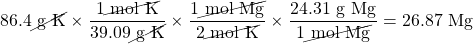# Test Yourself

What mass of H2 will be produced when 122 g of Zn are reacted?

Zn(s) + 2 HCl(aq) → ZnCl2(aq) + H2(g)

3.77 g

Key Takeaways

• Mole quantities of one substance can be related to mass quantities using a balanced chemical equation.
• Mass quantities of one substance can be related to mass quantities using a balanced chemical equation.
• In all cases, quantities of a substance must be converted to moles before the balanced chemical equation can be used to convert to moles of another substance.

Exercises

# Questions

1. What mass of CO2 is produced by the combustion of 1.00 mol of CH4?

CH4(g) + 2O2(g) → CO2(g) + 2H2O(ℓ)

2. What mass of H2O is produced by the combustion of 1.00 mol of CH4?

CH4(g) + 2O2(g) → CO2(g) + 2H2O(ℓ)

3. What mass of HgO is required to produce 0.692 mol of O2?

2HgO(s) → 2Hg(ℓ) + O2(g)

4. What mass of NaHCO3 is needed to produce 2.659 mol of CO2?

2NaHCO3(s) → Na2CO3(s) + H2O(ℓ) + CO2(g)

5. How many moles of Al can be produced from 10.87 g of Ag?

Al(NO3)3(s) + 3Ag → Al + 3AgNO3

6. How many moles of HCl can be produced from 0.226 g of SOCl2?

SOCl2(ℓ) + H2O(ℓ) → SO2(g) + 2HCl(g)

7. How many moles of O2 are needed to prepare 1.00 g of Ca(NO3)2?

Ca(s) + N2(g) + 3O2(g) → Ca(NO3)2(s)

8. How many moles of C2H5OH are needed to generate 106.7 g of H2O?

C2H5OH(ℓ) + 3O2(g) → 2CO2(g) + 3H2O(ℓ)

9. What mass of O2 can be generated by the decomposition of 100.0 g of NaClO3?

2NaClO3 → 2NaCl(s) + 3O2(g)

10. What mass of Li2O is needed to react with 1,060 g of CO2?

Li2O(aq) + CO2(g) → Li2CO3(aq)

11. What mass of Fe2O3 must be reacted to generate 324 g of Al2O3?

Fe2O3(s) + 2Al(s) → 2Fe(s) + Al2O3(s)

12. What mass of Fe is generated when 100.0 g of Al are reacted?

Fe2O3(s) + 2Al(s) → 2Fe(s) + Al2O3(s)

13. What mass of MnO2 is produced when 445 g of H2O are reacted?

H2O(ℓ) + 2MnO4(aq) + Br(aq) → BrO3(aq) + 2MnO2(s) + 2OH(aq)

14. What mass of PbSO4 is produced when 29.6 g of H2SO4 are reacted?

Pb(s) + PbO2(s) + 2H2SO4(aq) → 2PbSO4(s) + 2H2O(ℓ)

15. If 83.9 g of ZnO are formed, what mass of Mn2O3 is formed with it?

Zn(s) + 2MnO2(s) → ZnO(s) + Mn2O3(s)

16. If 14.7 g of NO2 are reacted, what mass of H2O is reacted with it?

3NO2(g) + H2O(ℓ) → 2HNO3(aq) + NO(g)

17. If 88.4 g of CH2S are reacted, what mass of HF is produced?

CH2S + 6F2 → CF4 + 2HF + SF6

18. If 100.0 g of Cl2 are needed, what mass of NaOCl must be reacted?

NaOCl + HCl → NaOH + Cl2

1. 44.0 g
1. 3.00 × 102 g
1. 0.0336 mol
1. 0.0183 mol
1. 45.1 g
1. 507 g
1. 4.30 × 103 g
1. 163 g
1. 76.7 g